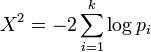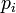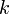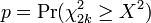# Combined p-Values$X^2 = -2\sum^k_{i=1} \log p_i$$p_i$ is the p-Value of the$i$th of$k$ tests, and$p = \Pr(\chi^2_{2k} \ge X^2)$